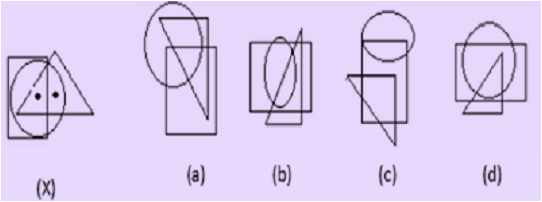# CREST Olympiads - CMO PDF Sample Papers for Class 8

Resources:

Class 8 sample paper & practice questions for CREST Mathematics Olympiad (CMO) level 1 are given below. Syllabus for level 1 is also mentioned for these exams. You can refer these sample paper & quiz for preparing for the CMO exam.Q.1 Q.2 Q.3 Q.4 Q.5 Q.6 Q.7 Q.8 Q.9 Q.10
 Q.1 Select the figure which satisfies the same conditions of placement of the dots as in fig. (X).a) a b) b c) c d) d
 Q.2 If in the word 'DISTURBANCE', the first letter is interchanged with the last letter, the second letter is interchanged with the tenth letter and so on, which letter would come after the letter T in the newly formed word? a) N b) C c) S d) E
 Q.3 Whole number such that twice of its square added to itself gives 10? a) 5 b) 2 c) 8 d) 10
 Q.4 For a fixed base, if the exponent decreases by 1, the number becomes: a) 1/10th of the previous number. b) 10 times of the previous number. c) Hundredth of the previous number. d) Hundredth times of the previous number.
 Q.5 A four-digit number aabb is divisible by 55. Then possible value(s) of b is/are: a) 0 and 2 b) 2 and 5 c) 0 and 5 d) Can't be determined
 Q.6 (a + 2b)² - (a + 2b)(3a - 7b) by factorisation gives: a) (a + 2b) (9b - 2a) b) a - 3b + 4ab c) 5b - 4a d) None of these
 Q.7 10 identical pipes fill water tank in 4 hours, 8 pipes fill same tank in: a) 6 hours b) 7 hours c) 5 hours d) 4 hours
 Q.8 Which is the smallest square number that is divisible by each of the number 4, 9 and10? a) 900 b) 810 c) 800 d) 920
 Q.9 If it is possible to from a word with the first, fourth, seventh and eleventh letters in the word 'SPHERVLVODS' write the second letter of that word. a) V b) L c) S d) E
 Q.10 The height of a rectangle in a histogram shows the _____________________. a) Width of the Class. b) Upper Limit of the Class. c) Lower limit of the Class. d) Frequency of the Class.Sample PDF of CREST Olympiads - CREST Mathematics Olympiad (CMO) PDF Sample Papers for Class 8: